## How to Calculate and Solve for Randomly Oriented Composite Modulus of Elasticity | Composites

The randomly oriented composite modulus of elasticity is illustrated by the image below.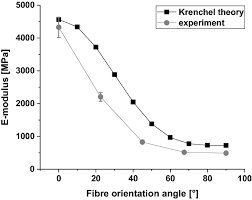To compute for randomly oriented composite modulus of elasticity, five essential parameters are needed and these parameters are Fibre Efficiency Parameter (K), Elastic Modulus of the Fibre (Ef), Elastic Modulus of the Matrix (Em), Volume Fraction of the Fibre (Vf) and Volume Fraction of the Matrix (Vm).

The formula for calculating randomly oriented composite modulus of elasticity:

E = KEfVf + EmVm

Where:

E = Randomly Oriented Composite Modulus of Elasticity
K = Fibre Efficiency Parameter
Ef = Elastic Modulus of the Fibre
Em = Elastic Modulus of the Matrix
Vf = Volume Fraction of the Fibre
Vm = Volume Fraction of the Matrix

Let’s solve an example;
Find the randomly oriented composite modulus of elasticity when the fibre efficiency parameter is 14, the elastic modulus of the fibre is 10, the elastic modulus of the matrix is 16, the volume fraction of the fibre is 12 and the volume fraction of the matrix is 8.

This implies that;

K = Fibre Efficiency Parameter = 14
Ef = Elastic Modulus of the Fibre = 10
Em = Elastic Modulus of the Matrix = 16
Vf = Volume Fraction of the Fibre = 12
Vm = Volume Fraction of the Matrix = 8

E = KEfVf + EmVm
E = (14)(10)(12) + (16)(8)
E = (1680) + (128)
E = 1808

Therefore, the randomly oriented composite modulus of elasticity is 1808 Pa.

## How to Calculate and Solve for Critical Fibre Strength for Composite | Composites

The critical fibre strength for composite is illustrated by the image below.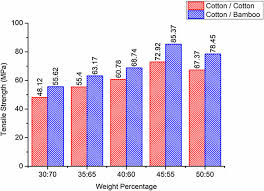To compute for critical fibre strength for composite, three essential parameters are needed and these parameters are Stress in Matrix at Fibre Failure (σ’m), Fibre Critical Tensile Strength (σ*f) and Volume Fraction of the Fibre (Vf).

The formula for calculating critical fibre strength for composite:

σ*a = σ’m(1 – Vf) + σ*fVf

Where:

σ*a = Critical Fibre Strength for Composite
σ’m = Stress in Matrix at Fibre Failure
σ*f = Fibre Critical Tensile Strength
Vf = Volume Fraction of the Fibre

Let’s solve an example;
Find the critical fibre strength for composite when the stress in matrix at fibre failure is 12, the fibre critical tensile strength is 10 and the volume fraction of the fibre is 9.

This implies that;

σ’m = Stress in Matrix at Fibre Failure = 12
σ*f = Fibre Critical Tensile Strength = 10
Vf = Volume Fraction of the Fibre = 9

σ*a = σ’m(1 – Vf) + σ*fVf
σ*a = 12(1 – 9) + (10)(9)
σ*a = 12(-8) + (90)
σ*a = (-96) + (90)
σ*a = -6

Therefore, the critical fibre strength for composite is -6 Pa.

## How to Calculate and Solve for Elastic Modulus on Transverse Direction | Composites

The elastic modulus on transverse direction is illustrated by the image below.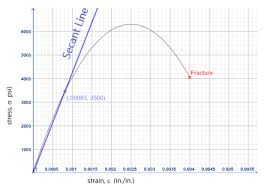To compute for elastic modulus on transverse direction, three essential parameters are needed and these parameters are Elastic Modulus of the Fibre (Ef), Elastic Modulus of the Matrix (Em) and Volume Fraction of the Fibre (Vf).

The formula for calculating elastic modulus on transverse direction:

Ec = EmEf/(1 – Vf)Ef + VfEm

Where:

Ec = Elastic Modulus on Transverse Direction
Em = Elastic Modulus of the Fibre
Ef = Elastic Modulus of the Matrix
Vf = Volume Fraction of the Fibre

Given an example;
Find the elastic modulus on transverse direction when the elastic modulus of the fibre is 4, the elastic modulus of the matrix is 8 and the volume fraction of the fibre is 6.

This implies that;

Em = Elastic Modulus of the Fibre = 4
Ef = Elastic Modulus of the Matrix = 8
Vf = Volume Fraction of the Fibre = 6

Ec = EmEf/(1 – Vf)Ef + VfEm
Ec = (4)(8)/(1 – 6)8 + (6)(4)
Ec = (32)/(-5)8 + (24)
Ec = (32)/(-40) + (24)
Ec = (32)/(-16)
Ec = -2

Therefore, the elastic modulus on transverse direction is -2 Pa.

## How to Calculate and Solve for Ratio of Load of Fibre to Matrix | Composites

The ratio of load of fibre to matrix is illustrated by the image below.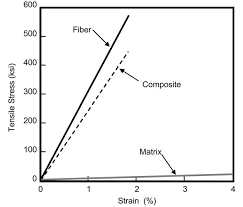To compute for ratio of load of fibre to matrix, four essential parameters are needed and these parameters are Elastic Modulus of the Fibre (Ef), Elastic Modulus of the Matrix (Em), Volume Fraction of the Fibre (Vf) and Volume Fraction of the Matrix (Vm).

The formula for calculating ratio of load of fibre to matrix:

F(f/m) = EfVf/EmVm

Where:

F(f/m) = Ratio of Load of Fibre to Matrix
Ef = Elastic Modulus of the Fibre
Em = Elastic Modulus of the Matrix
Vf = Volume Fraction of the Fibre
Vm = Volume Fraction of the Matrix

Let’s solve an example;
Find the ratio of load of fibre to matix when the elastic modulus of the fibre is 14, the elastic modulus of the matrix is 10, the volume fraction of the fibre is 6 and the volume fraction of the matrix is 2.

This implies that;

Ef = Elastic Modulus of the Fibre = 14
Em = Elastic Modulus of the Matrix = 10
Vf = Volume Fraction of the Fibre = 6
Vm = Volume Fraction of the Matrix = 2

F(f/m) = EfVf/EmVm
F(f/m) = (14)(6)/(10)(2)
F(f/m) = (84)/(20)
F(f/m) = 4.2

Therefore, the ratio of load of fibre to matrix is 4.2.

Calculating the Elastic Modulus of the Fibre when the Ratio of Load of Fibre to Matrix, the Elastic Modulus of the Matrix, the Volume Fraction of the Fibre and the Volume Fraction of the Matrix is Given.

Ef = F(f/m) x EmVm / Vf

Where:

Ef = Elastic Modulus of the Fibre
F(f/m) = Ratio of Load of Fibre to Matrix
Em = Elastic Modulus of the Matrix
Vf = Volume Fraction of the Fibre
Vm = Volume Fraction of the Matrix

Let’s solve an example;
Find the elastic modulus of the fibre when the ratio of load of fibre to matrix is 20, the elastic modulus of the matrix is 4, the volume fraction of the matrix is 2 and the volume fraction of the fibre is 12.

This implies that;

F(f/m) = Ratio of Load of Fibre to Matrix = 20
Em = Elastic Modulus of the Matrix = 4
Vf = Volume Fraction of the Fibre = 12
Vm = Volume Fraction of the Matrix = 2

Ef = F(f/m) x EmVm / Vf
Ef = 20 x (4)(2) / 12
Ef = 20 x 8 / 12
Ef = 160 / 12
Ef = 13.33

Therefore, the elastic modulus of the fibre is 13.33

## How to Calculate and Solve for Elastic Modulus on Longitudinal Direction | Composites

The elastic modulus on longitudinal direction is illustrated by the image below.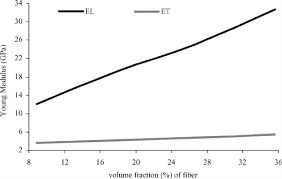To compute for elastic modulus on longitudinal direction, three essential parameters are needed and these parameters are Elastic Modulus of the Matrix (Em), Elastic Modulus of the Fibre (Ef) and Volume Fraction of the Fibre (Vf).

The formula for calculating elastic modulus on longitudinal direction:

Eu = Em(1 – Vf) + EfVf

Where:

Eu = Elastic Modulus on Longitudinal Direction
Em = Elastic Modulus of the Matrix
Ef = Elastic Modulus of the Fibre
Vf = Volume Fraction of the Fibre

Let’s solve an example;
Find the elastic modulus on longitudinal direction when the elastic modulus of the matrix is 12, the elastic modulus of the fibre is 10 and volume fraction of the fibre is 4.

This implies that;

Em = Elastic Modulus of the Matrix = 12
Ef = Elastic Modulus of the Fibre = 10
Vf = Volume Fraction of the Fibre = 4

Eu = Em(1 – Vf) + EfVf
Eu = (12)(1 – 4) + (10)(4)
Eu = (12)(-3) + (40)
Eu = (-36) + (40)
Eu = 4

Therefore, the elastic modulus on longitudinal direction is 4 Pa.

## How to Calculate and Solve for Total Composite Strength | Composites

The total composite strength is illustrated by the image below.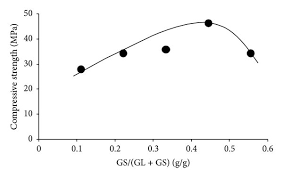To compute for total composite strength, four essential parameters are needed and these parameters are Strength of the Matrix (σm), Strength of the Fibre (σf), Volume Fraction of the Matrix (Vm) and Volume Fraction of the Fibre (Vf).

The formula for calculating total composite strength:

σc = σmVm + σfVf

Where:

σc = Total Composite Strength
σm = Strength of the Matrix
σf = Strength of the Fibre
Vm = Volume Fraction of the Matrix
Vf = Volume Fraction of the Fibre

Let’s solve an example;
Find the total composite strength when the strength of the matrix is 6, the strength of the fibre is 12, the volume fraction of the matrix is 10 and the volume fraction of the fibre is 4.

This implies that;

σm = Strength of the Matrix = 6
σf = Strength of the Fibre = 12
Vm = Volume Fraction of the Matrix = 10
Vf = Volume Fraction of the Fibre = 4

σc = σmVm + σfVf
σc = (6)(10) + (12)(4)
σc = (60) + (48)
σc = 108

Therefore, the total composite strength is 108 Pa.

Calculating the Strength of the Matrix when the Total Composite Strength, the Strength of the Fibre, the Volume Fraction of the Matrix and the Volume Fraction of the Fibre is Given.

σm = σc – σfVf / Vm

Where:

σm = Strength of the Matrix
σc = Total Composite Strength
σf = Strength of the Fibre
Vm = Volume Fraction of the Matrix
Vf = Volume Fraction of the Fibre

Let’s solve an example;
Find the strength of the matrix when the total composite strength is 40, the strength of the fibre is 10, the volume fractions of the matrix is 5 and the volume fractions of the fibre is 2.

This implies that;

σc = Total Composite Strength = 40
σf = Strength of the Fibre = 10
Vm = Volume Fraction of the Matrix = 5
Vf = Volume Fraction of the Fibre = 2

σm = σc – σfVf / Vm
σm = 40 – (10)(2) / 5
σm = 40 – 20 / 5
σm = 20 / 5
σm = 4

Therefore, the strength of the matrix is 4.

## How to Calculate and Solve for Total Load on Composite | Composites

The total load on composite is illustrated by the image below.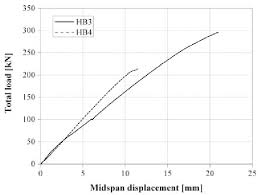To compute for total load on composite, two essential parameters are needed and this parameters are Load on Matrix (Fm) and Load on Fibre (Ff).

The formula for calculating total load on composite:

Fc = Fm + Ff

Where:

Fc = Total Load on Composite

Let’s solve an example;
Find the total load on composite when the load on matrix is 20 and the load on fibre is 10.

This implies that;

Fm = Load on Matrix = 20
Ff = Load on Fibre = 10

Fc = Fm + Ff
Fc = (20) + (10)
Fc = 30

Therefore, the total load on composite is 30.

Calculating the Load on Matrix when the Total Load on Composite and the Load on Fibre is Given.

Fm = Fc – Ff

Where:

Fc = Total Load on Composite

Let’s solve an example;
Find the load on matrix when the total load on composite is 34 and the load on fibre is 12.

This implies that;

Fc = Total Load on Composite = 34
Ff = Load on Fibre = 12

Fm = Fc – Ff
Fm = 34 – 12
Fm = 22

Therefore, the load on matrix is 22

## How to Calculate and Solve for Critical Fibre Length | Composites

The critical fibre length is illustrated by the image below.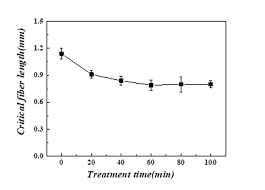To compute for critical fibre length, three essential parameters are needed and these parameters are Critical Fibre Stress (σ*f), Fibre Diameter (d) and Shear Yield Strength (τc).

The formula for calculating critical fibre length:

lc = σ*fd/2τc

Where:

lc = Critical Fibre Length
σ*f = Critical Fibre Stress
d = Fibre Diameter
τc = Shear Yield Strength

Let’s solve an example;
Find the critical fibre length when the critical fibre stress is 15, the fibre diameter is 3 and the shear yield strength is 10.

This implies that;

σ*f = Critical Fibre Stress = 15
d = Fibre Diameter = 3
τc = Shear Yield Strength = 10

lc = σ*fd/2τc
lc = (15)(3)/2(10)
lc = (45)/(20)
lc = 2.25

Therefore, the critical fibre length is 2.25 cm.

Calculating the Critical Fibre Stress when the Critical Fibre Length, the Fibre Diameter and the Shear Yield Strength is Given.

σ*f = lc x 2τc / d

Where:

σ*f = Critical Fibre Stress
lc = Critical Fibre Length
d = Fibre Diameter
τc = Shear Yield Strength

Let’s solve an example;
Given the critical fibre length as 24, the fibre diameter as 12 and the shear yield strength as 4. Find the critical fibre stress?

This implies that;

lc = Critical Fibre Length = 24
d = Fibre Diameter = 12
τc = Shear Yield Strength = 4

σ*f = lc x 2τc / d
σ*f = 24 x 2(4) / 12
σ*f = 24 x 8 / 12
σ*f = 192 / 12
σ*f = 16

Therefore, the critical fibre stress is 16.

## How to Calculate and Solve for Modulus of Elasticity of Composites Lower Bound | Composites

The modulus of elasticity of composites lower bound is illustrated by the image below.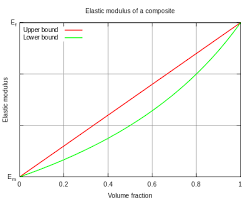To compute for modulus of elasticity of composites lower bound, four essential parameters are needed and these parameters are Modulus of Elasticity of the Matrix (Em), Modulus of Elasticity of the Particle (Ep), Volume Fraction of the Matrix (Vm) and Volume Fraction of the Particle (Vp).

The formula for calculating modulus of elasticity of composites lower bound:

Ec(l) = EmEp/VmEp + VpEm

Where:

Ec(u) = Modulus of Elasticity of Composites Lower Bound
Em =Modulus of Elasticity of the Matrix
Ep = Modulus of Elasticity of the Particle
Vm = Volume Fractions of the Matrix
Vp = Volume Fractions of the Particle

Let’s solve an example;
Find the modulus of elasticity of composites lower bound when the modulus of elasticity of the matrix is 2, the modulus of elasticity of the particle is 6, the volume fractions of the matrix is 8 and the volume fractions of the particle is 4.

This implies that;

Em =Modulus of Elasticity of the Matrix = 2
Ep = Modulus of Elasticity of the Particle = 6
Vm = Volume Fractions of the Matrix = 8
Vp = Volume Fractions of the Particle = 4

Ec(l) = EmEp/VmEp + VpEm
Ec(l) = (2)(6)/(8)(6) + (4)(2)
Ec(l) = (12)/(48) + (8)
Ec(l) = (12)/(56)
Ec(l) = 0.214

Therefore, the modulus of elasticity of composites lower bound is 0.214 Pa.

## How to Calculate and Solve for Modulus of Elasticity of Composites Upper Bound | Composites

The modulus of elasticity of composites upper bound is illustrated by the image below.To compute for modulus of elasticity of composites upper bound, four essential parameters are needed and these parameters are Modulus of Elasticity of the Matrix (Em), Modulus of Elasticity of the Particle (Ep), Volume Fraction of the Matrix (Vm) and Volume Fraction of the Particle (Vp).

The formula for calculating modulus of elasticity of composites upper bound:

Ec(u) = EmVm + EpVp

Where:

Ec(u) = Modulus of Elasticity of Composites Upper Bound
Em =Modulus of Elasticity of the Matrix
Ep = Modulus of Elasticity of the Particle
Vm = Volume Fractions of the Matrix
Vp = Volume Fractions of the Particle

Let’s solve an example;
Find the modulus of elasticity of composites upper bound when the modulus of elasticity of the matrix is 4, the modulus of elasticity of the particle is 7, the volume fractions of the matrix is 2 and the volume fractions of the particle is 6.

This implies that;

Em =Modulus of Elasticity of the Matrix = 4
Ep = Modulus of Elasticity of the Particle = 7
Vm = Volume Fractions of the Matrix = 2
Vp = Volume Fractions of the Particle = 6

Ec(u) = EmVm + EpVp
Ec(u) = (4)(2) + (7)(6)
Ec(u) = (8) + (42)
Ec(u) = 50

Therefore, the modulus of elasticity of composites upper bound is 50 Pa.

Calculating the Modulus of Elasticity of the Matrix when the Modulus of Elasticity of Composites Upper Bound, the Modulus of Elasticity of the Particle, the Volume Fraction of the Matrix and the Volume Fraction of the Particle is Given.

Em = Ec(u) – EpVp / Vm

Where:

Em =Modulus of Elasticity of the Matrix
Ec(u) = Modulus of Elasticity of Composites Upper Bound
Ep = Modulus of Elasticity of the Particle
Vm = Volume Fractions of the Matrix
Vp = Volume Fractions of the Particle

Let’s solve an example;
Find the modulus of elasticity of the matrix when the modulus of elasticity of composites upper bound is 40, the modulus of elasticity of the particle is 10, the volume fractions of the matrix is 5 and the volume fractions of the particle is 2.

This implies that;

Ec(u) = Modulus of Elasticity of Composites Upper Bound = 40
Ep = Modulus of Elasticity of the Particle = 10
Vm = Volume Fractions of the Matrix = 5
Vp = Volume Fractions of the Particle = 2

Em = Ec(u) – EpVp / Vm
Em = 40 – (10)(2) / 5
Em = 40 – 20 / 5
Em = 20 / 5
Em = 4

Therefore, the modulus of elasticity of the matrix is 4.